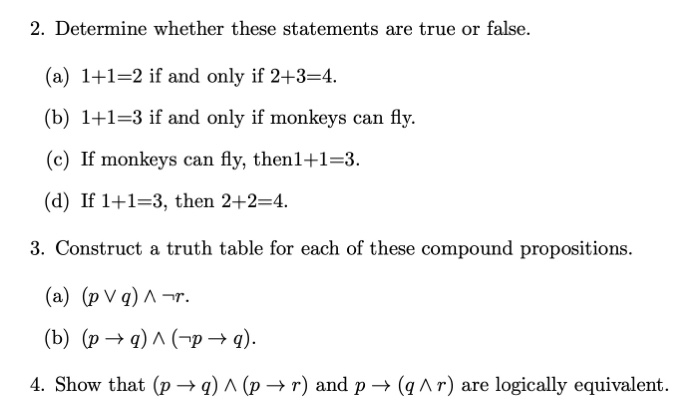# Question 2. Determine whether these statements are true or false. (a) 1+1=2 if and only if 2+3=4. (b) 1+1=3 if and only if monkeys can fly. (c) If monkeys can fly, then1+1=3. (d) If 1+1=3, then 2+2=4. 3. Construct a truth table for each of these compound propositions. (a) (pVq) A-s. (b) (p +9) ^ ( p + q). 4. Show that (p +9)^(p+r) and p + (qar) are logically equivalent.NHMIJR The Asker · Other MathematicsTranscribed Image Text: 2. Determine whether these statements are true or false. (a) 1+1=2 if and only if 2+3=4. (b) 1+1=3 if and only if monkeys can fly. (c) If monkeys can fly, then1+1=3. (d) If 1+1=3, then 2+2=4. 3. Construct a truth table for each of these compound propositions. (a) (pVq) A-s. (b) (p +9) ^ ( p + q). 4. Show that (p +9)^(p+r) and p + (qar) are logically equivalent.
More
Transcribed Image Text: 2. Determine whether these statements are true or false. (a) 1+1=2 if and only if 2+3=4. (b) 1+1=3 if and only if monkeys can fly. (c) If monkeys can fly, then1+1=3. (d) If 1+1=3, then 2+2=4. 3. Construct a truth table for each of these compound propositions. (a) (pVq) A-s. (b) (p +9) ^ ( p + q). 4. Show that (p +9)^(p+r) and p + (qar) are logically equivalent.